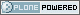# Departamento de Matematica

##### Personal tools
You are here: Home » Coloquios

# Coloquios del departamento 2019

Próxima charla: Bob Oliver "Local structure of finite groups and of their classifying spaces." - Jueves 14 de marzo de 2019 - 15h - Aula de seminario nuevo del DM/IMAS.Coloquios del Departamento de Matemática 2019

## Local structure of finite groups and of their classifying spaces.

##### Resumen:

Let p be a prime. By the p-local structure of a finite group G is meant a Sylow p-subgroup S and the G-conjugacy relations among the subgroups of S. More precisely, two finite groups G and H are said to be p-locally equivalent (have the same p-local structure) if there is an isomorphism S->T for some S in Syl_p(G) and T in Syl_p(H), that preserves all G- and H-conjugacy relations among subgroups of S and of T. A classifying space of a finite group G is an Eilenberg-MacLane space of type K(G; 1); i.e., a topological space BG whose fundamental group is isomorphic to G and whose universal covering space is contractible. Two classifying spaces BG and BH are said to be p-locally equivalent if there is a third space X, and maps f:BG->X and f':BH->X, such that f and f' both induce isomorphisms in homology with coefficients in Z/p. A conjecture by Martino and Priddy, now a theorem, says that for each pair of finite groups G and H and each prime p, G and H are p-locally equivalent if and only if their classifying spaces are p-locally equivalent. This was first proven by me, but only by assuming the classification of finite simple groups and doing a long, messy case-by-case analysis involving those groups. Later, a much simpler proof was found by Chermak, but still assuming the classification of finite simple groups, and recently Glauberman and Lynd succeeded in modifying Chermak’s argument to get a classification-free proof. In the talk, I want to first describe in more detail the background of this theorem, and also talk very briefly about some of the ideas behind its proof. I’ll then give an application: a purely group theoretic statement whose only known proof is based on homotopy theory. If there’s time left, I’ll then describe a related result involving automorphisms of groups and of their classifying spaces, and give a few more examples and applications involving finite simple groups.

Created by nsaintie